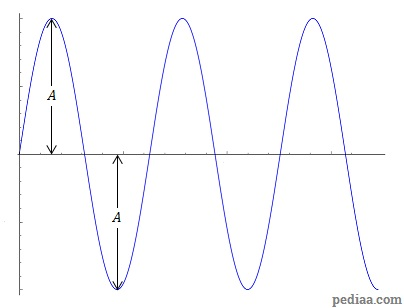# Difference Between Amplitude and Magnitude

## Main Difference – Amplitude vs. Magnitude

Amplitude and magnitude are both terms used to describe properties of quantities. The main difference between amplitude and magnitude is that amplitude refers to the furthest values that a quantity can take from 0 whereas magnitude refers to the size of a quantity regardless of direction.

## What is Amplitude

The term amplitude describes the maximum and minimum values reached by a periodically changing quantity. Typically, the term is used with quantities that vary sinusoidally. In this context, amplitude refers to the maximum deviation from the equilibrium. i.e. if the quantity$x$ can be described with an equation in the form of$x(t)=A\mathrm{sin\:}2\pi ft$, then$A$ is the amplitude. The diagram below illustrates this:For a sinusoidally-varying quantity, the amplitude refers to the maximum and minimum values taken by the quantity.

When the amplitude$A$ of a wave is proportional to the wave’s energy, the intensity of the wave (that is, the power transmitted by the wave per unit area) is proportional to the square of its amplitude. When we describe simple harmonic motion, amplitude refers to the maximum displacement of the oscillating object from the equilibrium position.

## What is Magnitude

Magnitude refers to the size of a quantity, regardless of the quantity’s direction (if the quantity has one). For a vector$\vec{a}$ of$n$ dimensions$\vec{a}=\left( a_1,a_2,a_3,\cdots a_n \right)$, the magnitude$\left| \vec{a}\right|$ is given by:$\sqrt{{a_1}^2+{a_2}^2+\cdots +{a_3}^2}$

Since no direction is associated with a magnitude, the magnitudes of vector quantities are scalar quantities. For instance, the magnitude of displacement is the distance, and the magnitude of velocity is speed, both of which are scalars.

Magnitude is also the of a unit in astronomy that measures the brightness of astronomical objects. Earthquakes are described using “magnitudes” along the Ritcher scale.

## Difference Between Amplitude and Magnitude

Amplitude refers to the maximum deviation from zero that can be taken by a periodically varying quantity.

Magnitude refers to the size of a quantity regardless of the direction.